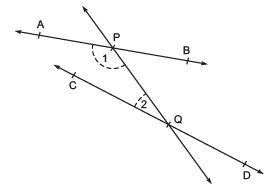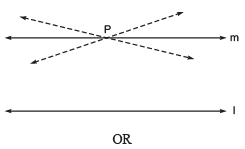Courses

# Short Notes - Introduction to Euclid’s Geometry Class 9 Notes | EduRev

## Class 9 Mathematics by Full Circle

Created by: Full Circle

## Class 9 : Short Notes - Introduction to Euclid’s Geometry Class 9 Notes | EduRev

The document Short Notes - Introduction to Euclid’s Geometry Class 9 Notes | EduRev is a part of the Class 9 Course Class 9 Mathematics by Full Circle.
All you need of Class 9 at this link: Class 9

Facts that Matter

• The unproved universal truths are called axioms or postulates.
• Theorems are statements which can be proved using definitions and axioms.
• Euclid’s postulates are:
(i) A straight line may be drawn from any one point to any other point.
(ii) A terminated line can be produced indefinitely.
(iii) A circle can be drawn with any centre and radius
(iv) All right angles are equal to one another.
(v) If a straight line falling on two straight lines makes the interior angles on the same side of it taken together less than two right angles, then the two straight lines, if produced indefinitely, meet on the side on which the sum of angles is less than two right angles.
• Playfair’s Axiom For every line ‘ℓ’ and for every point ‘P’ not lying on ℓ’, there exists a unique line passing through ‘P’ and parallel to ‘ℓ’’.

EUCLID’S DEFINITIONS, AXIOMS AND POSTULATES
Euclid was a Greek mathematician, who introduced the method of proving a geometrical result by using logical reasonings on previously proved and known results.
Statement is a sentence which can be judged to be true or false.
A Theorem is a statement which requires a proof.
Note: Proving a theorem means estabilishing the truth of that theorem.
Corollary is a statement whose truth can easily be deduced from a theorem.
Axioms are the basic facts which are taken for granted without proof.
Postulates are the basic facts which are taken for granted specific to geometry, without proof.

Some of the Euclid’s axioms are:
(i) The whole is greater than the part.
(ii) Things which are double of the same things are equal to one another.
(iii) Things which are halves of the same things are equal to one another.
(iv) Things which are equal to the same thing are equal to one another.
(v) Things which coincide with one another are equal to one another.
(vi) If equals are added to equals, the wholes are equal.
(vii ) If equals are subtracted from equals, the remainders are equal.
Note: (i) Euclidean Geometry is the study of flat surface.
(ii) Now-a-days ‘postulates’ and ‘axioms’ are terms that are used interchangeably and in the same sense.

EUCLID’S FIVE POSTULATES
Postulate 1: A straight line may be drawn from any point to any other point.
Postulate 2: A terminated line can be produced indefinitely.
Postulate 3: A circle can be drawn with any centre and any radius.
Postulate 4: All right angles are equal to one another.
Postulate 5: If a straight line falling on two straight lines makes the interior angles on the same side of it taken together is less than two right angles, then the two straight lines, if produced indefinitely, meet on that side on which the sum of the angles is less than two right angles.
Example. The straight line PQ falls on AB and CD such that the sum of the interior angles 1 and 2 is less than 180º on the left side of PQ. Thus, the lines AB and CD will intersect (on producing) on the left side of PQ.EQUIVALENT VERSIONS OF EUCLID’S FIFTH POSTULATE
In the history of mathematics, the Euclid’s fifth postulate is very significant. Though there are several equivalent versions of this postulate, but we state the following axiom (called the playfair’s axiom) here: “For every line ‘l’ and for every point ‘P’ not lying on l, there exists a unique line m passing through P and parallel to l”Two distinct intersecting lines cannot be parallel to the same line.

132 docs

,

,

,

,

,

,

,

,

,

,

,

,

,

,

,

,

,

,

,

,

,

;